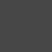# How to Calculate Percentage Score in Google Forms QuizPublished

onLearn how to find quiz score percentages in Google Forms using Google Sheets formulas.

You can create a simple 3-question quiz on Google Forms and earn 10 points for each correct answer. The maximum score that can be achieved in the quiz thus 30 points.

When someone takes the quiz and submits the form, the responses are recorded in the Google Sheets that is set as the response destination for the Google Form.

There is also something interesting here. If the associated form is a quiz, Google Sheets automatically adds a column to the answer sheet titled Score, which contains the total points scored by the respondent on the quiz.

Convert quiz score to percentage

Teachers may want to calculate the percentage scores students get on a quiz and assign grades accordingly. This can easily be done with an array formula in Google Sheets, but before we get there let’s see how to convert a quiz score (e.g. 20/30) to a percentage .

There are at least three ways to extract the quiz score from cell B2. Let’s look at some of them.

The REGEXREPLACE function replaces a string value that matches RegEx with another value. Here we start with the first character in the cell that is not a number, match everything until the end of the string and replace it with a blank. So the slash (/) and everything after the slash are replaced, leaving just the score.

=REGEXREPLACE(TO_TEXT(B2),”\D.+\$”,””)

The second method uses the SPLIT function to split the score column text with slashes as delimiters, and the INDEX function to get the first value in the split array containing the scores.

The following approach uses the SEARCH function to locate the slash in the cell and the LEFT function to get everything before the slash.

=LEFT(B2,SEARCH(“/”,B2)-1)

A similar approach can be used to get the maximum quiz score. Its number is after the slash in the score column.

=REGEXREPLACE(TO_TEXT(B2),”\d.+/”,””) =INDEX(SPLIT(B2,”/”),2) =RIGHT(B2,SEARCH(“/”,B2)-1) calculation Percentage of quizzes

Now that we have formulas that extract the quiz score and total score separately, we can combine them to get a percentage score.

Options are:

=REGEXREPLACE(TO_TEXT(B2),”\D.+\$”,””)/REGEXREPLACE(TO_TEXT(B2),”\d.+/”,””) =INDEX(SPLIT(B2,”/”), 1)/INDEX(SPLIT(B2,”/”),2) =LEFT(B2,SEARCH(“/”,B2)-1)/RIGHT(B2,SEARCH(“/”,B2)-1)

Right-click the score column and from the context menu[左に 1 列挿入]and paste one of the above formulas into cell C2. You can then copy the formula to other rows containing quiz answers.

Automatically copy quiz score percentages

One of the drawbacks of the previous approach is that the formula has to be added to the row each time a new quiz is submitted.

A simple workaround for this issue is a formula copy approach that automatically adds formulas each time a new quiz form is submitted.

Go to cell C1 and paste the following formula:

=ArrayFormula(IF(ROW(B:B)=1, “Percentage”, IF(NOT(ISBLANK(B:B)),LEFT(B:B,SEARCH(“/”,B:B)-1)/ RIGHT(B:B,SEARCH(“/”,B:B)-1),)))

Look at the row index and if it’s the first row add the column title. Then check if column B has a score value and calculate the percentage score.

Then select column C and[書式]>[数値]>[パーセント]to format the calculated percentages appropriately.

You can also send a certificate based on your quiz score from Document Studio.